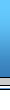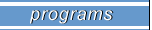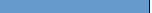- Alaska Home Loans - Buy A Home in Alaska - Refinance Your Loan - Mortgage Process - Nationwide Lenders - FIND A HOME - FIND A LOAN - Alaska Loans Articles - Contact Us- 97% Alaska home loans - 100% Alaska home loans - 103% Alaska home loans - VA home loans - FHA home loans - Alaska Jumbo Mortgages - Alaska Lot loans - Alaska 0 Down Loans - Conventional Loans

The most common buydown is the 2-1 buydown. In the past, for a buyer to secure a 2-1 buydown they would pay 3 points above current market points in order to pay a below market interest rate during the first two years of the loan. At the end of the two years they would then pay the old market rate for the remaining term.

As an example, if the current market rate for a conforming fixed rate loan is 8.5% at a cost of 1.5 points, the buydown gives the borrower a first year rate of 6.50%, a second year rate of 7.50% and a third through 30th year rate of 8.50% and the cost would be 4.5 points. Buydown were usually paid for by a transferring company because of the high points associated with them.

In today's market, mortgage companies have designed variations of the old buydowns rather than charge higher points to the buyer in the beginning they increase the note rate to cover their yields in the later years.

As an example, if the current rate for a conforming fixed rate loan is 8.50% at a cost of 1.5 points, the buydown would give the buyer a first year rate of 7.25%, a second year rate of 8.25% and a third through 30th year rate of 9.25% , or a three-quarter point higher note rate than the current market and the cost would remain at 1.5 points.

Another common buydown is the 3-2-1 buydown which works much in the same ways as the 2-1 buydown, with the exception of the starting interest rate being 3% below the note rate. Another variation is the flex-fixed buydown programs that increase at six month interval rather than annual intervals.

As an example, for a flex-fixed jumbo buydown at a cost of 1.5 points, the first six months rate would be 7.50%, the second six months the rate would be 8.00%, the next six months rate would be 8.50%, the next six months rate would be 9.00%, the next six months the rate would be 9.50% and at the 37th month the rate would reach the note rate of 9.875% and would remain there for the remainder of the term. A comparable jumbo 30 year fixed at 1.5 points would be 8.875%.

California Home Loans - Mortgage Leads - real estate web hosting